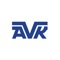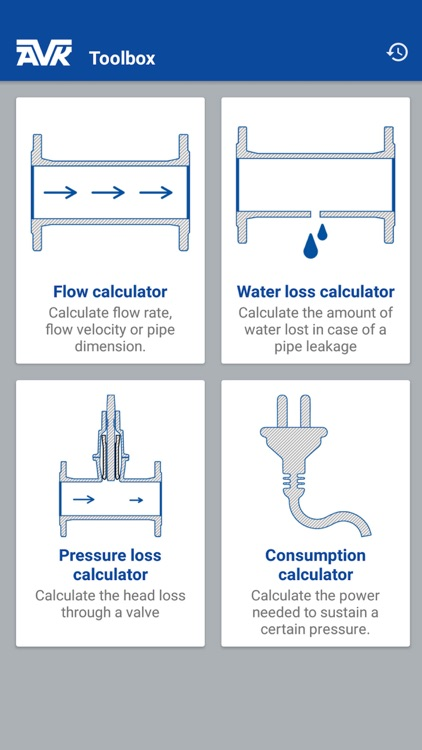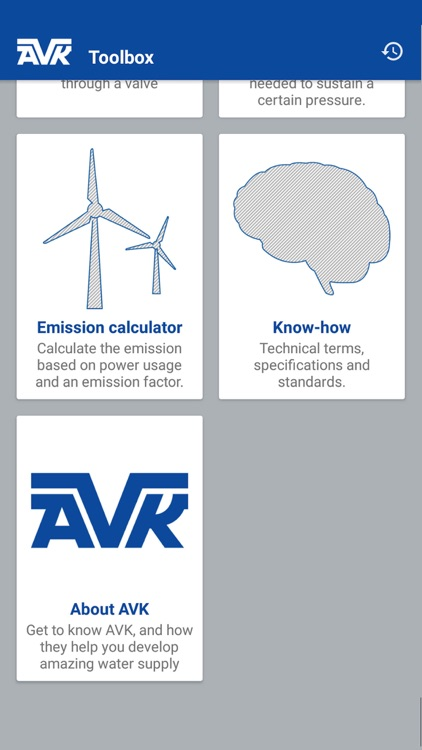You are using an outdated browser. Please upgrade your browser to improve your experience.The AVK app is a tool, both for internal and external useAVK Toolbox

by AVK Holding A/SWhat is it about?

The AVK app is a tool, both for internal and external use. The variety of functions will be described here in this guide. The calculating functions are:App Details

Version
2.0.2
Rating
NA
Size
39Mb
Genre
Last updated
March 17, 2019
Release date
September 23, 2016

App Store Description

The AVK app is a tool, both for internal and external use. The variety of functions will be described here in this guide. The calculating functions are:

- Flow calculator
- Water loss calculator
- Pressure loss calculator
- Power consumption calculator
- Emission calculator

The flow calculator needs two out of three inputs to make the calculator calculate the third – DN-class, flow velocity or flow rate. It doesn’t matter which of the three you type. If you give the input of flow velocity and flow rate, the DN-class will be calculated, but in a nominal size. The flow calculator knows the standard DN-classes and will therefor suggest a standard size and make you adjust the flow velocity or the flow rate, up or down.

The water loss calculator is calculating the amount of water lost in a pipe, in case of a leakage. The water loss calculator needs two inputs, the pressure of the pipe and the hole diameter. When these two inputs has been typed, the amount of water lost per hour, day and year is calculated.

The pressure loss calculator is calculating the pressure loss in a specific valve to a given flow rate. Therefor the pressure loss calculator needs the input of the flow rate and the valve flow factor (Kv-value). The valve flow factor can be typed as an arbitrary value, or by the use of the AVK valve picker. In the AVK valve picker a variety of AVK valves is present, with Kv-values to each specific valve series and DN-class. The pressure loss is calculated in bars.

The power consumption calculator is calculating the corresponding power consumption to a pressure loss at a given flow rate. Therefor the power consumption calculator needs the input of the flow rate, pressure difference and an efficiency grade for a pump. The efficiency grade is 0,80 as default. When the input is typed the power consumption is calculated in the amount of kWh/yr.

The emission calculator is calculating the CO2 emission from the power consumption at a given energy source. The emission calculator needs the input of the power consumption, in the amount of kWh/yr, and the emission factor, in the amount of g*CO2eq/kWh. The emission factor can be typed as an arbitrary value or an energy source can be picked from the app. In the energy source picker nine different energy sources will appear. It is possible to pick one energy source and set it for 100% of the energy, or divide the amount of energy on more than one energy source, by setting the percent relation between them.

Every time a calculation has been made, it is saved in the calculation history. The calculation history will be available in the top-right corner on the front page, or in each of the calculators in the top-right corner under history. Some of the calculators is connected to each other, in a way where it is possible to use one calculation in another calculation, by finding it in the calculation history and press insert. For instance if a calculation is made in the flow calculator, it is possible to use the result in the pressure loss calculator.

In the AVK toolbox it is possible to export the calculations as a PDF-file to an E-mail address. Open the calculation history and press share, then select your E-mail program, and send the mail to the desired E-mail address.
Besides these calculating features, the AVK toolbox will also bring a know-how feature where different technical information will appear. The last feature of the app, is an about AVK feature. Here the AVK history will appear and some other useful information.

Disclaimer:
AppAdvice does not own this application and only provides images and links contained in the iTunes Search API, to help our users find the best apps to download. If you are the developer of this app and would like your information removed, please send a request to [email protected] and your information will be removed.